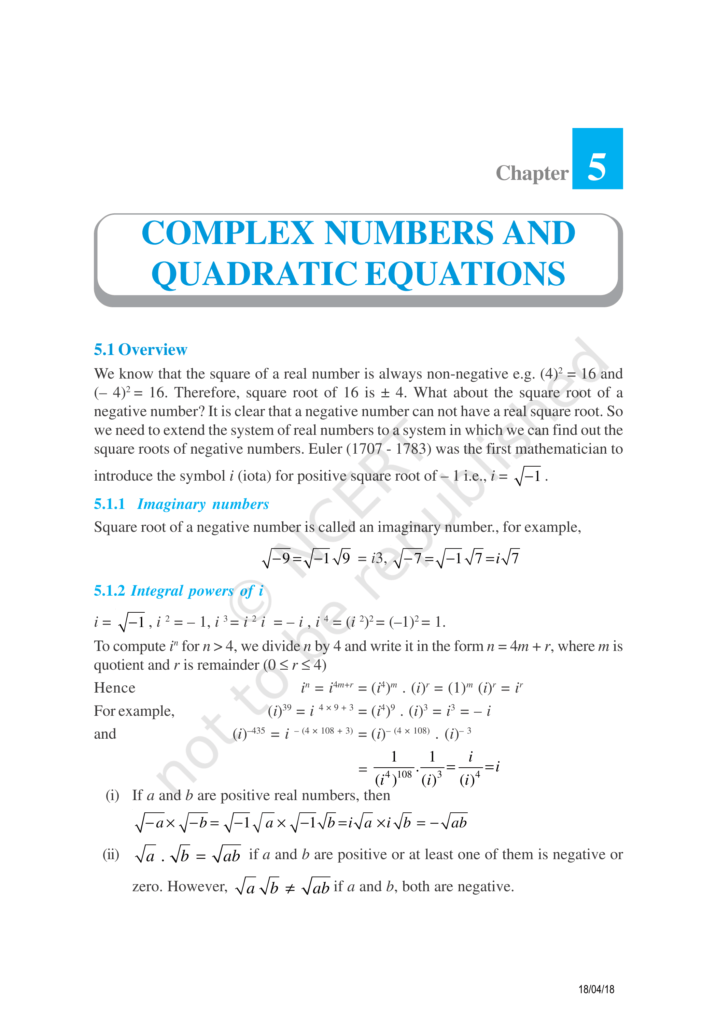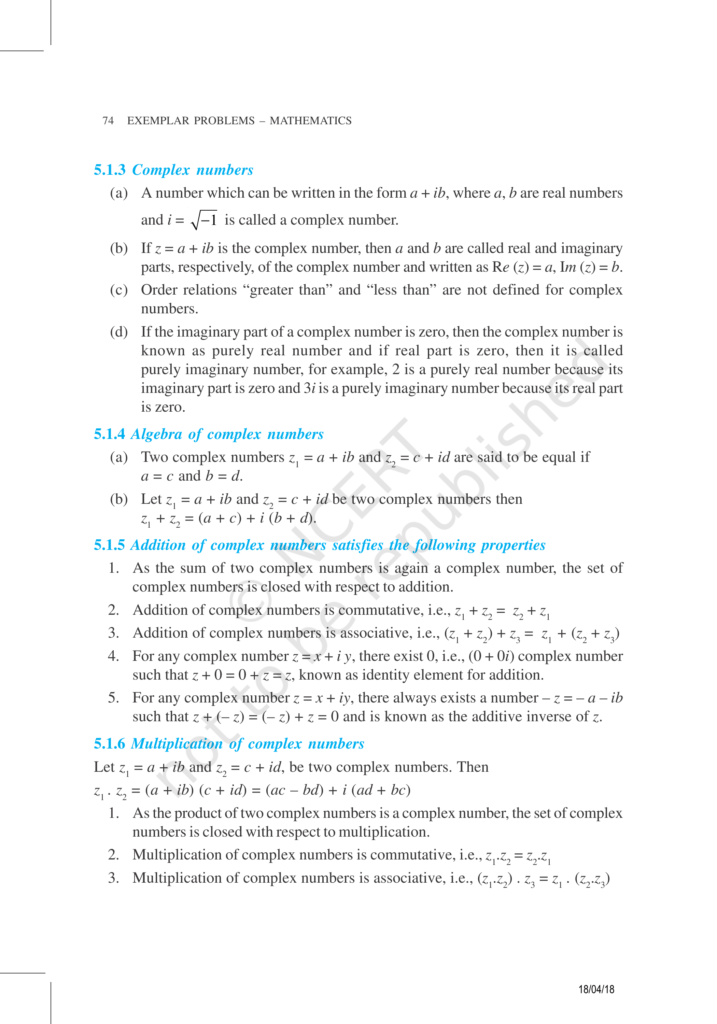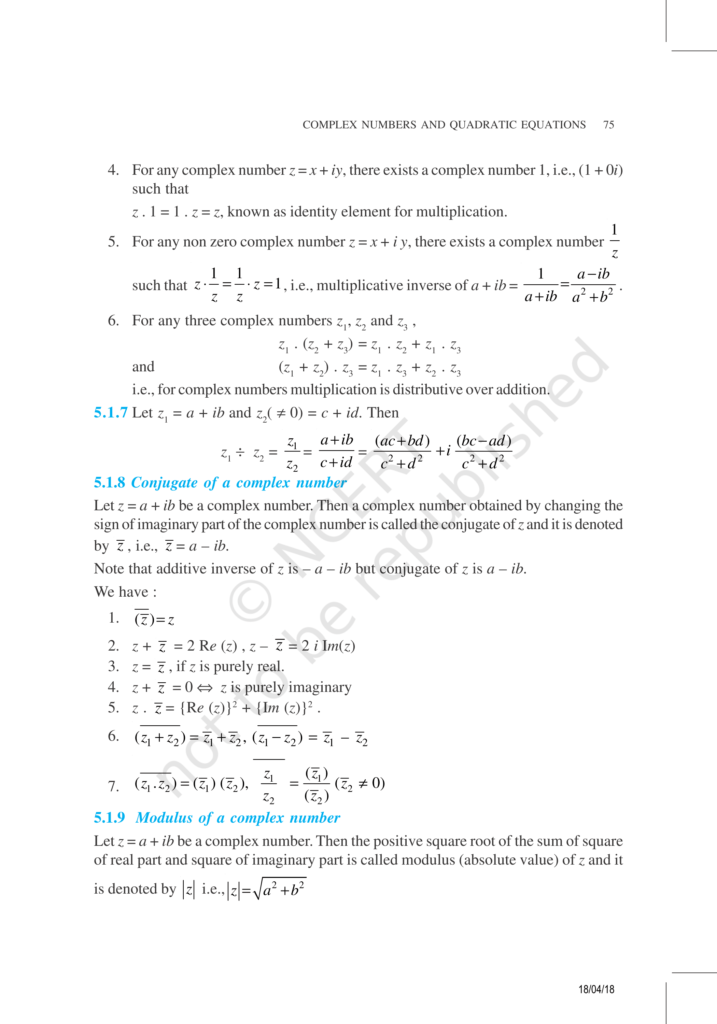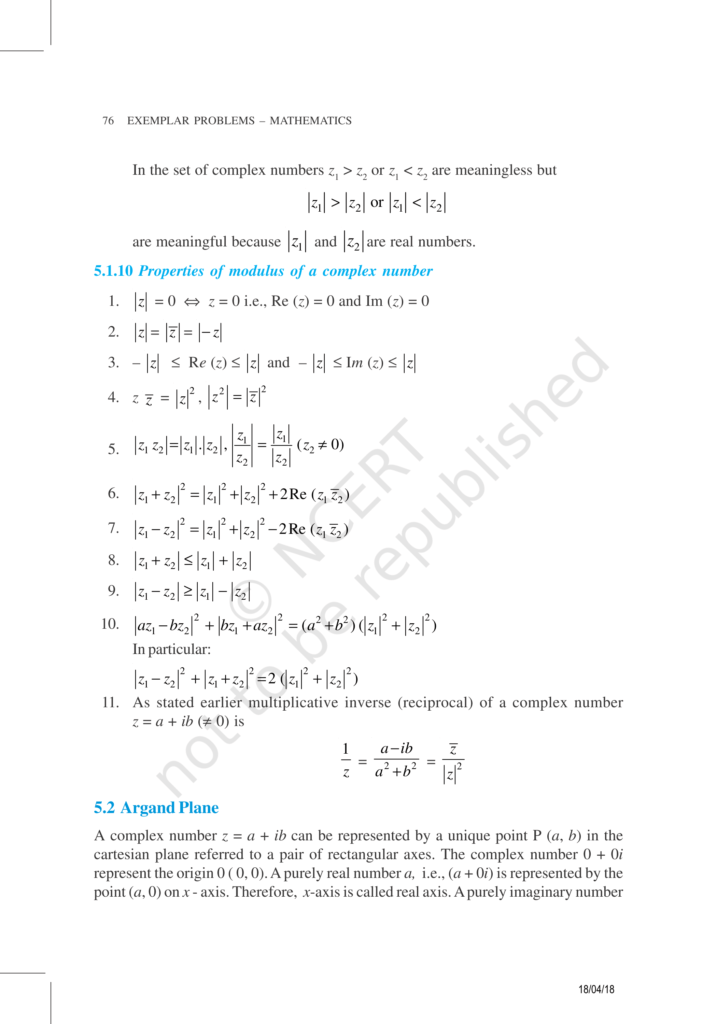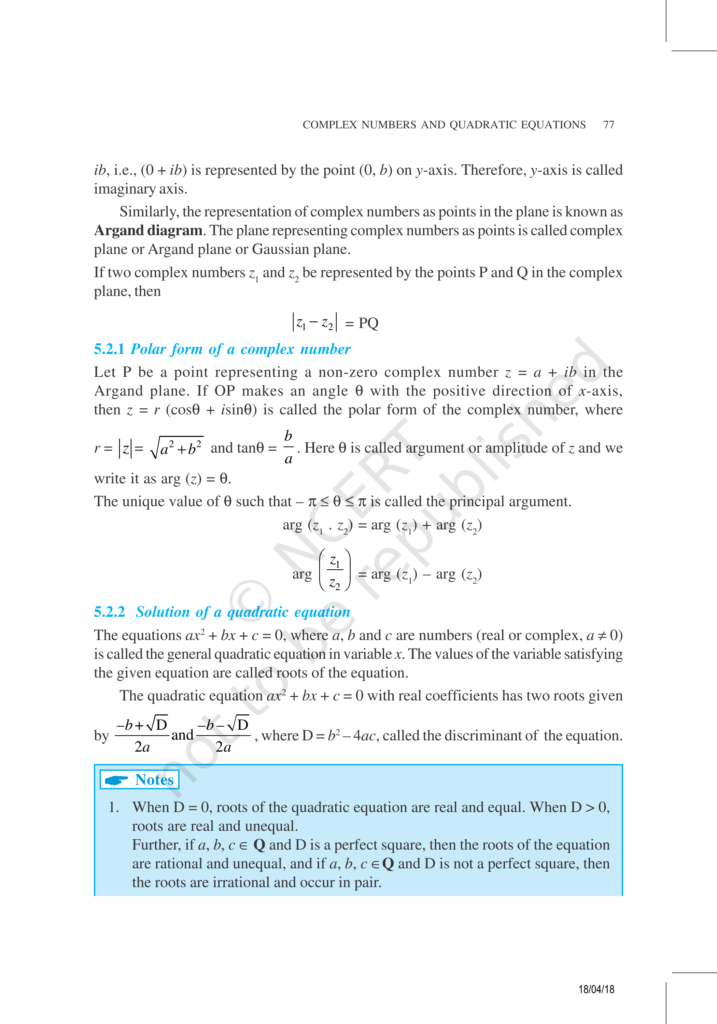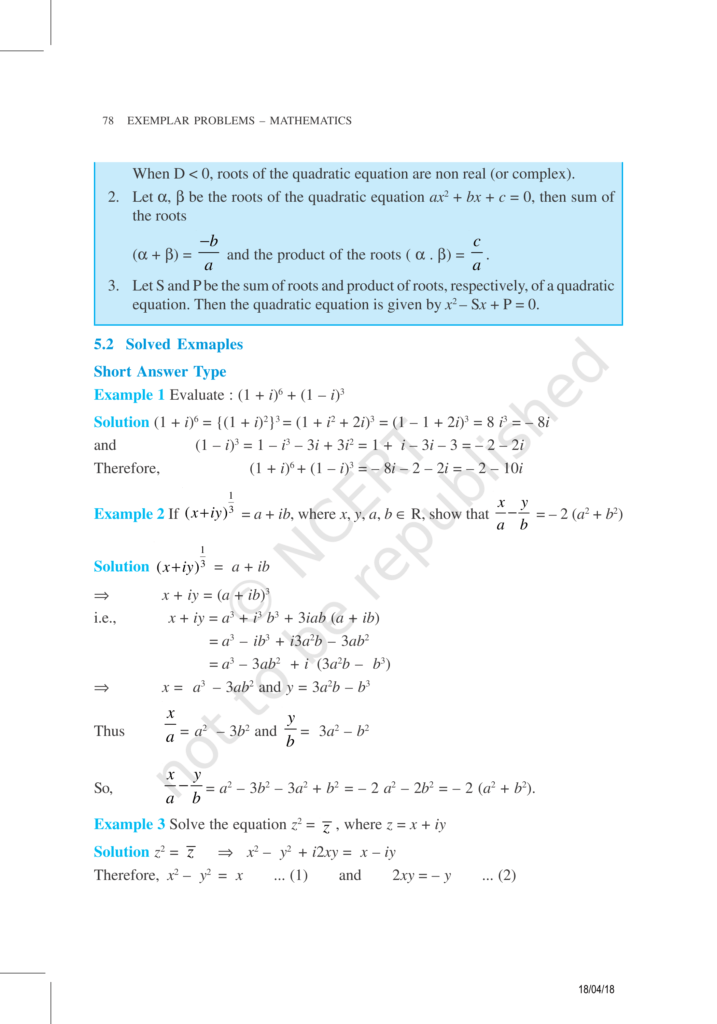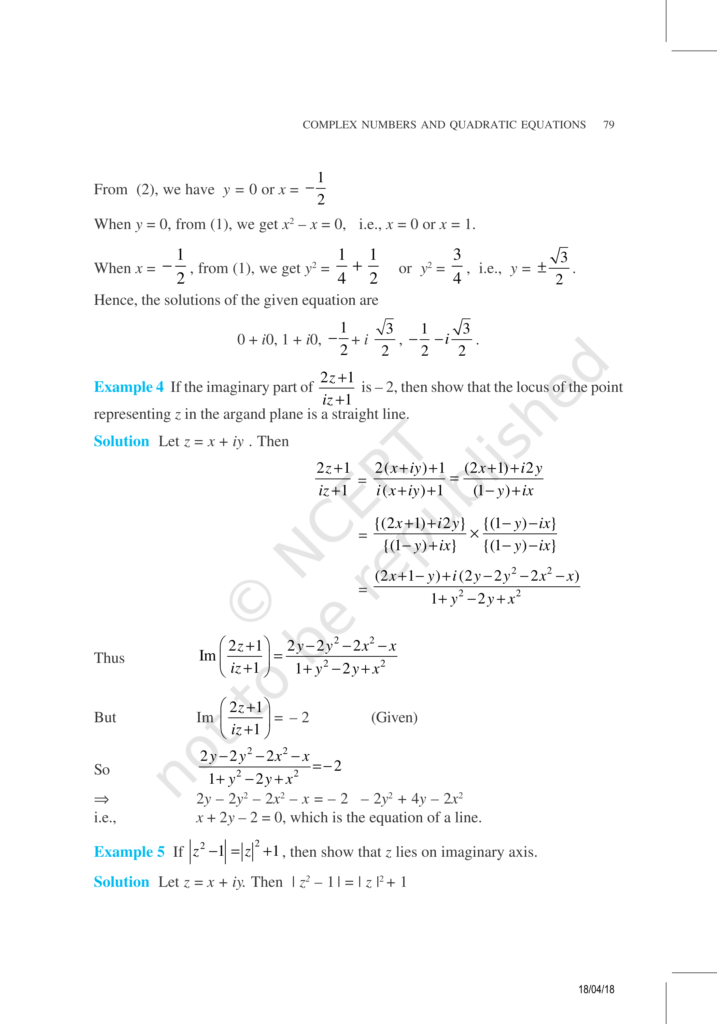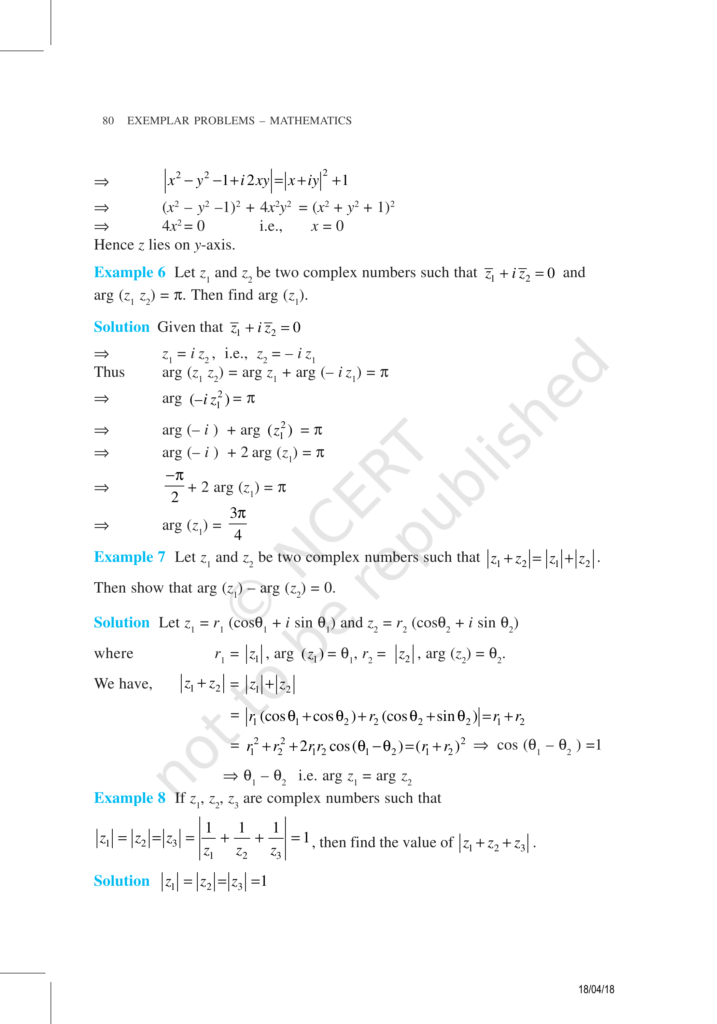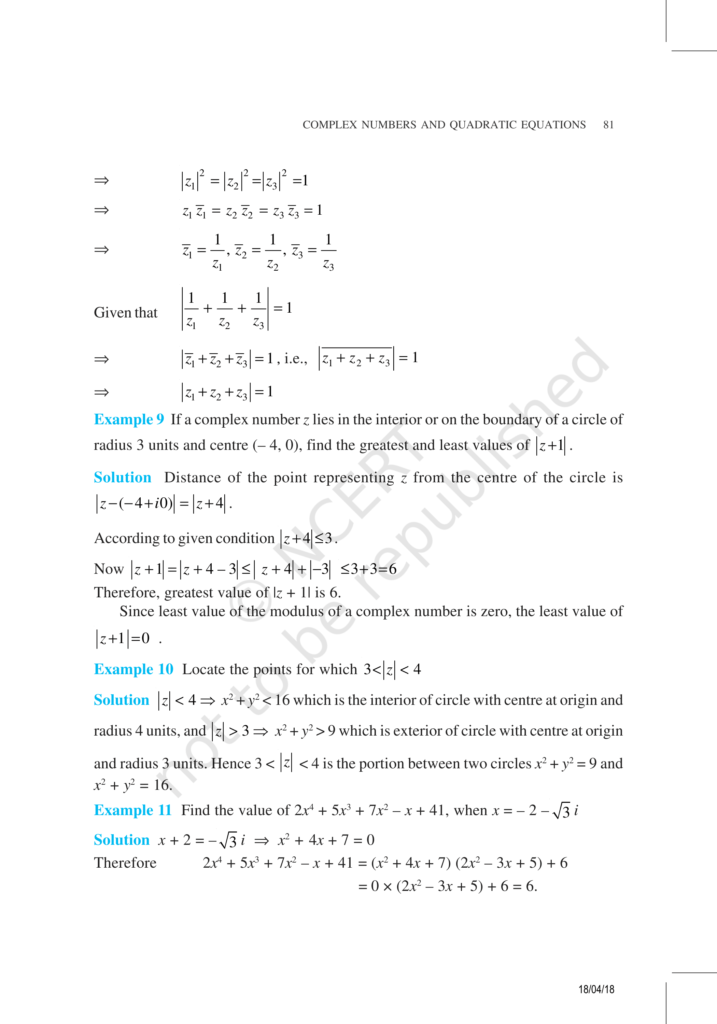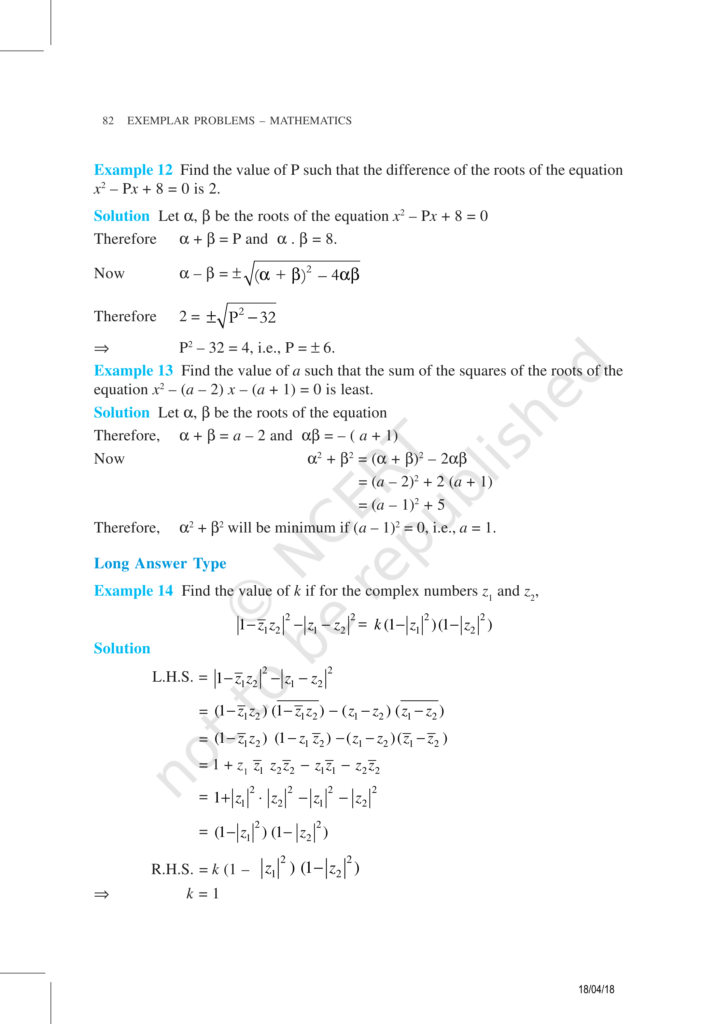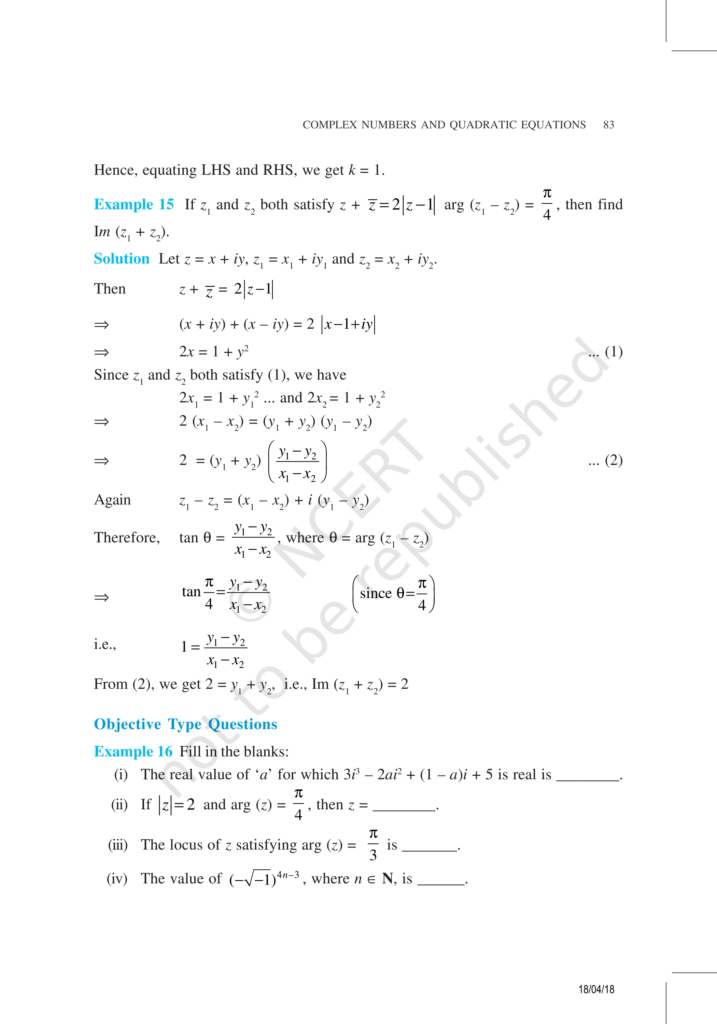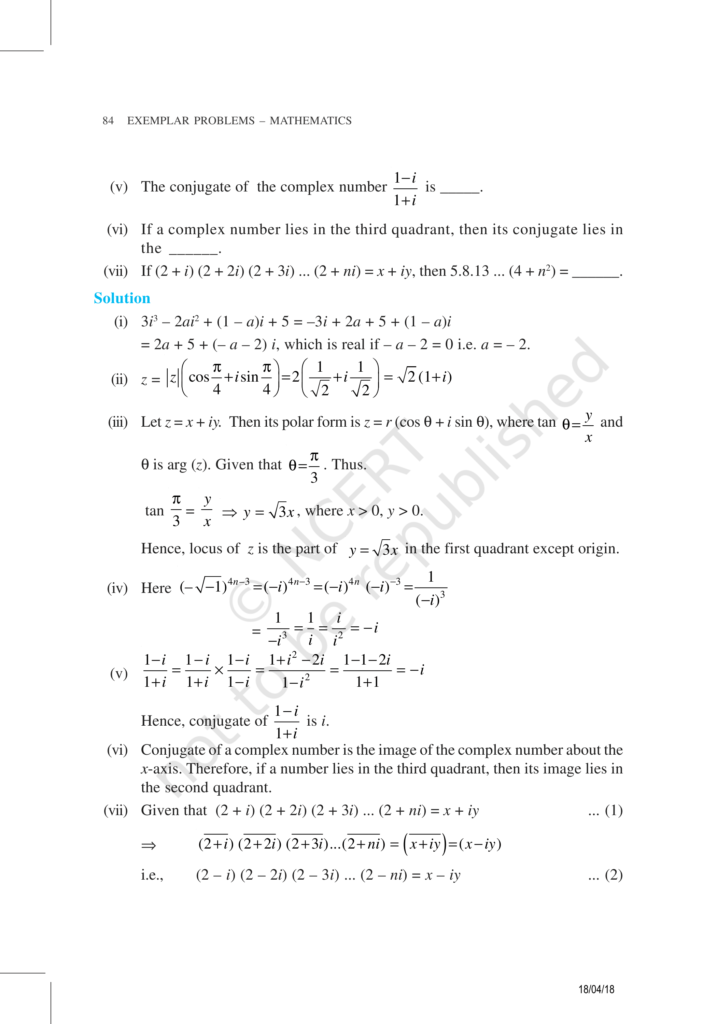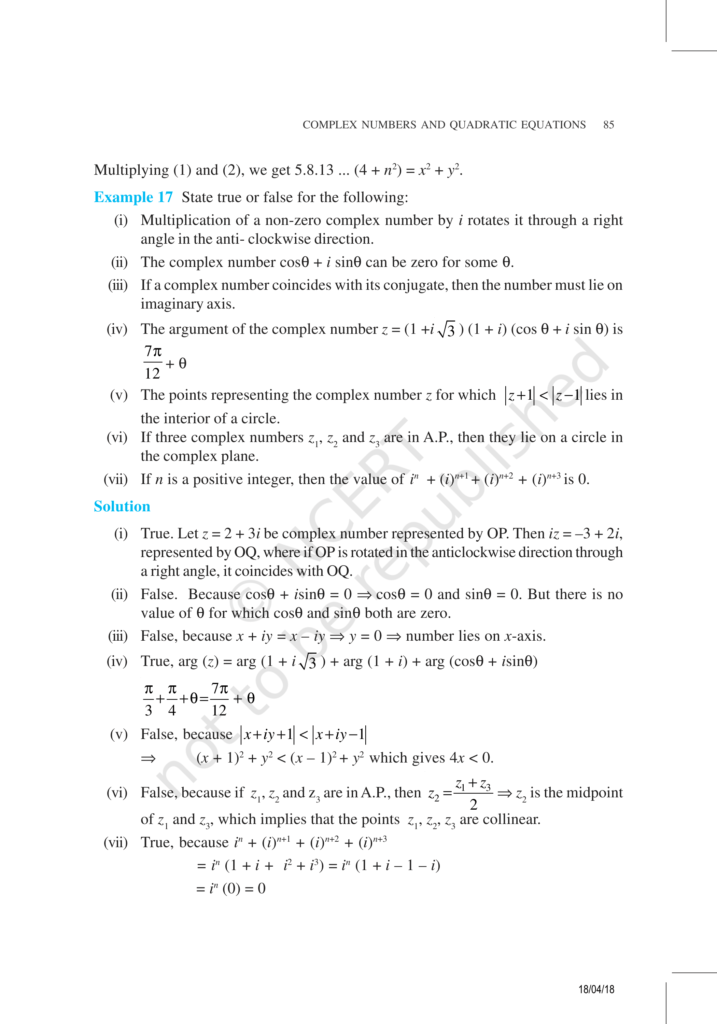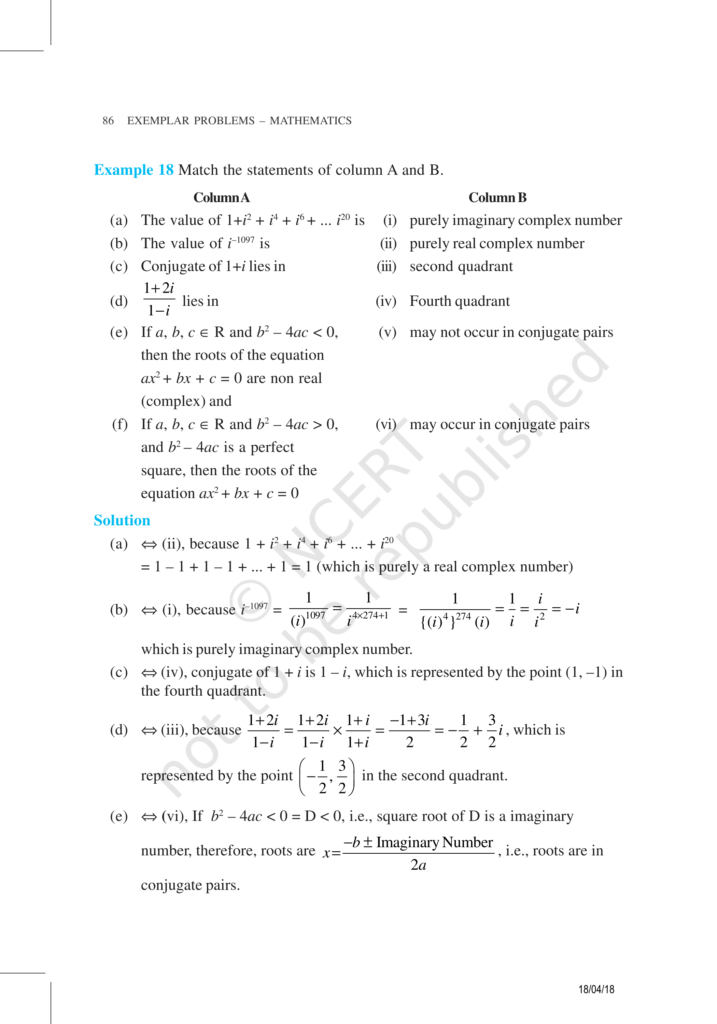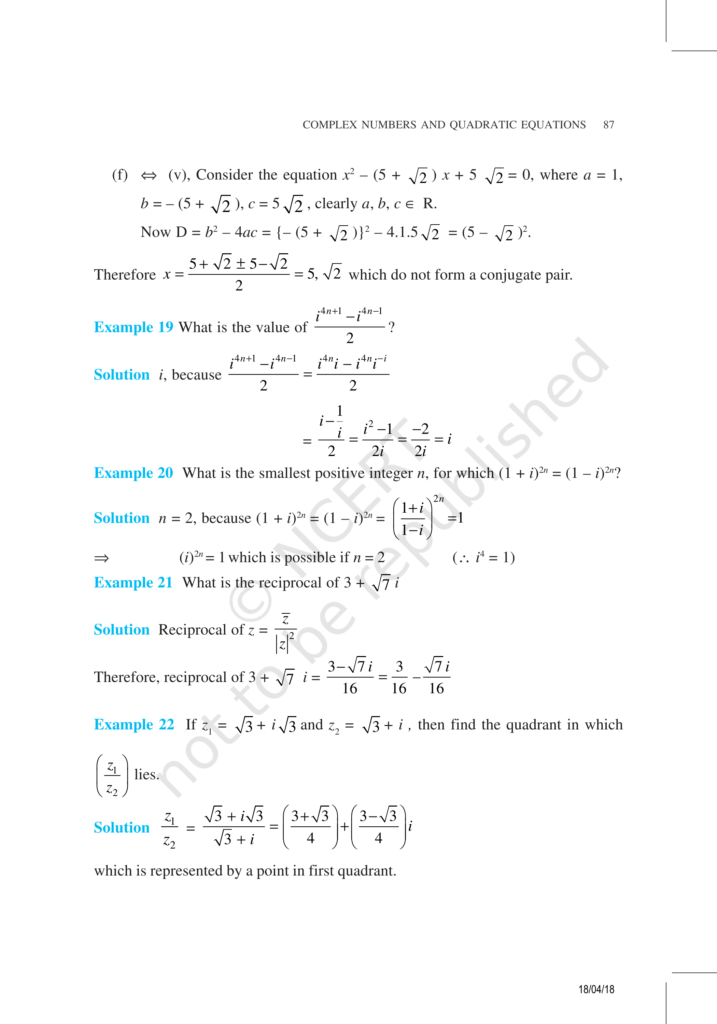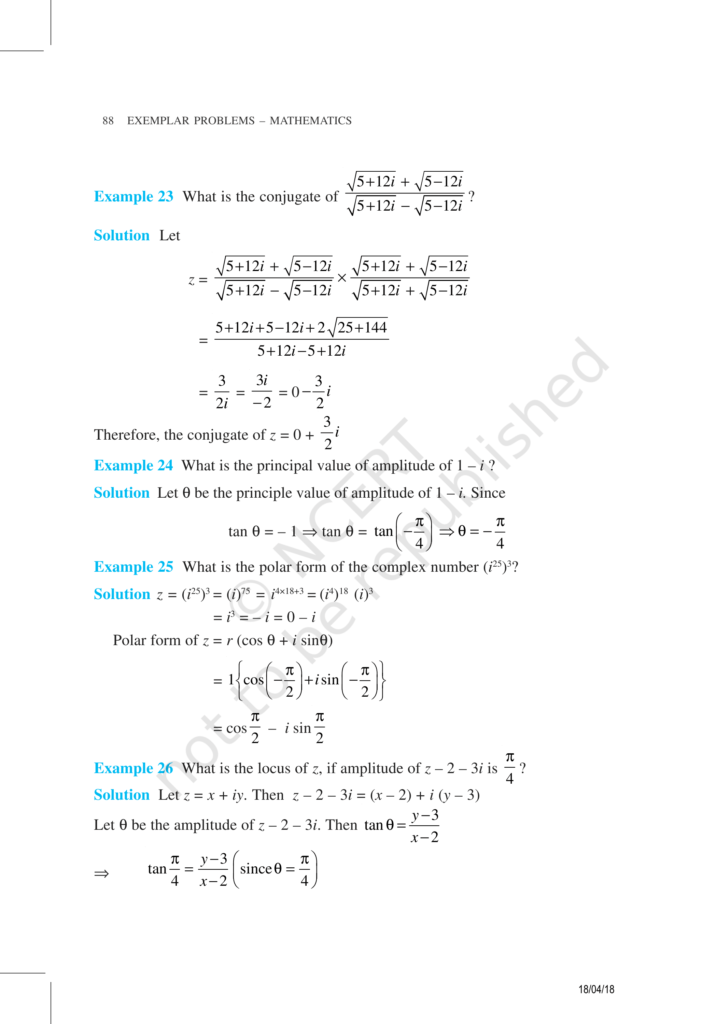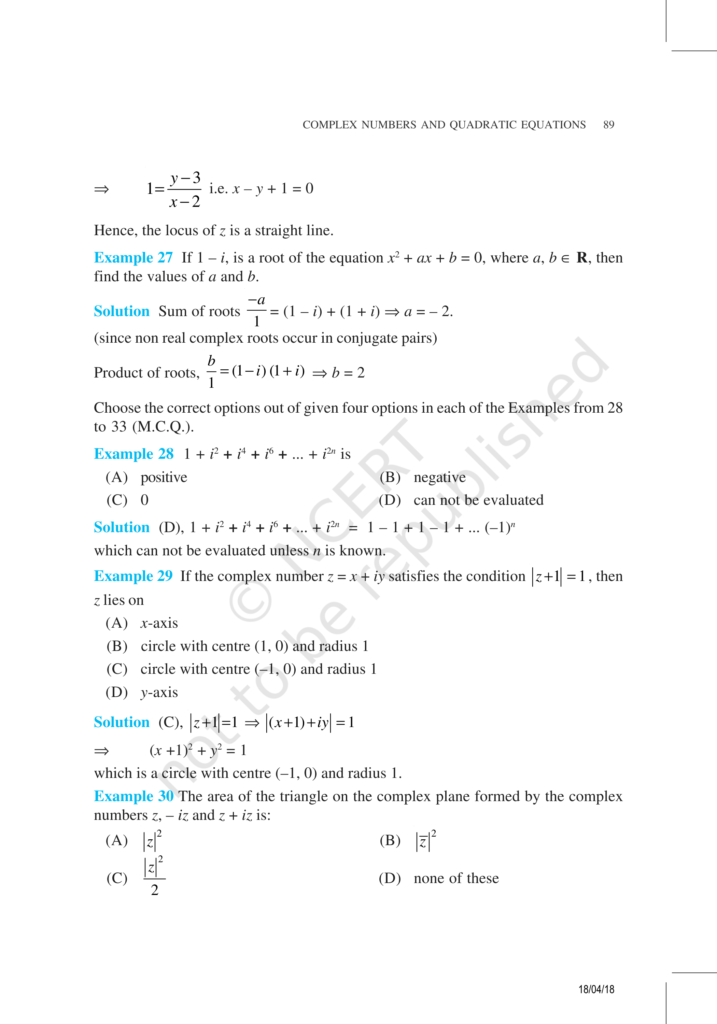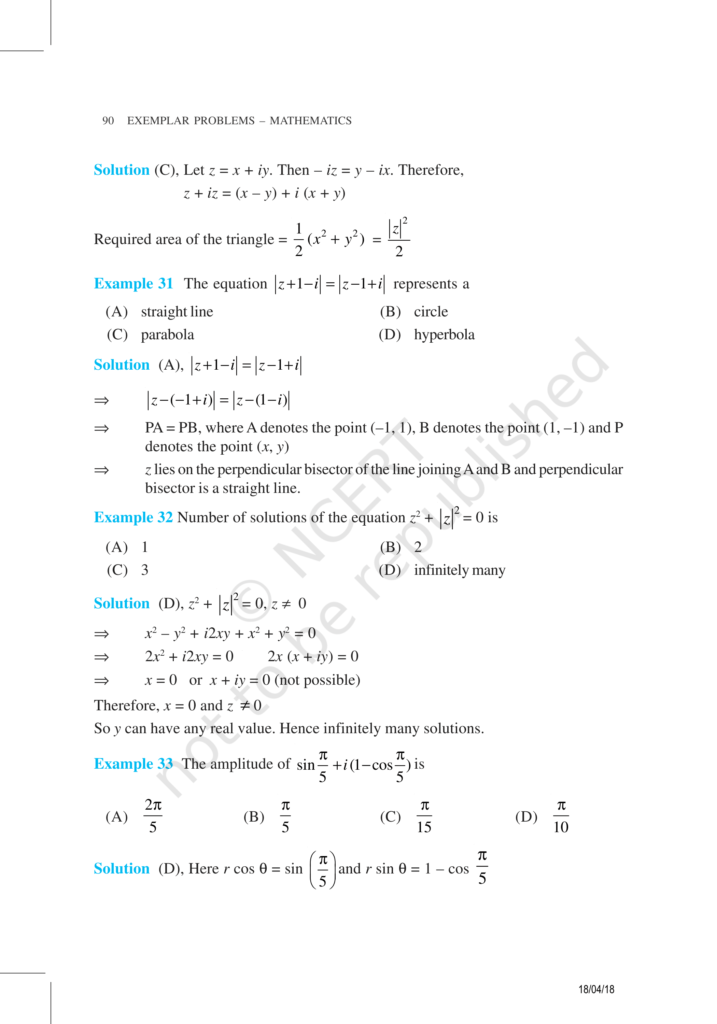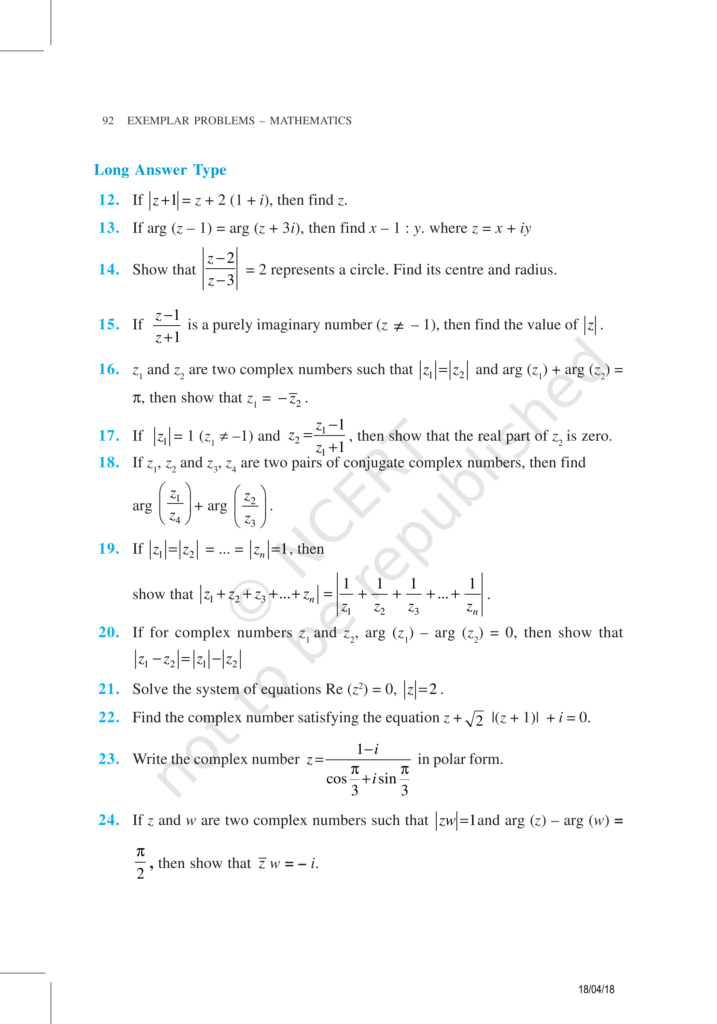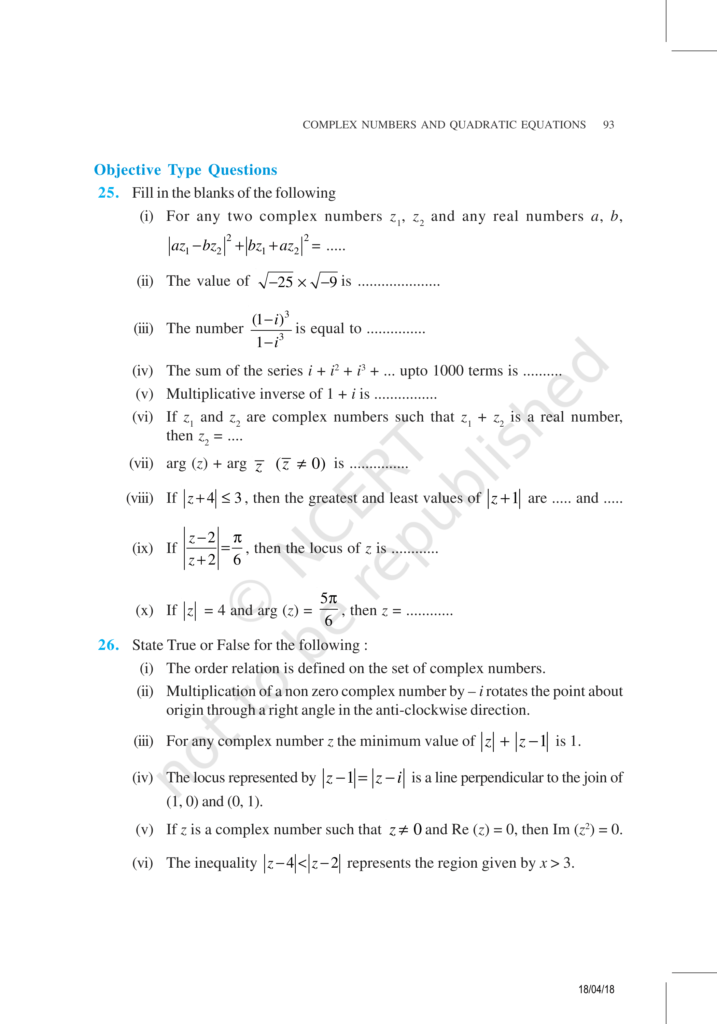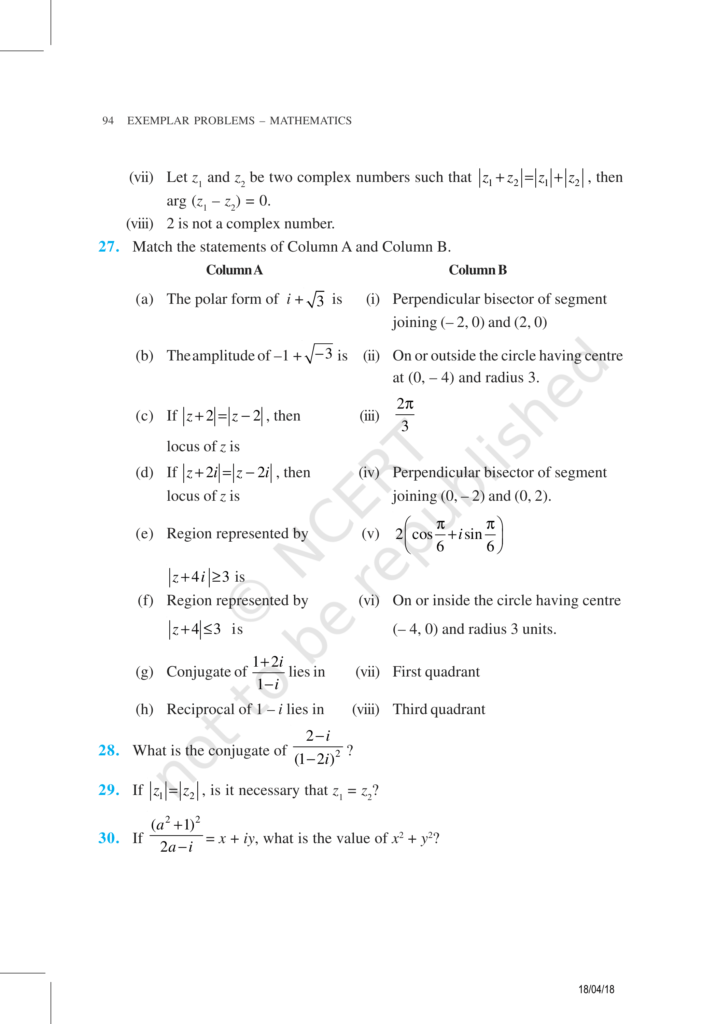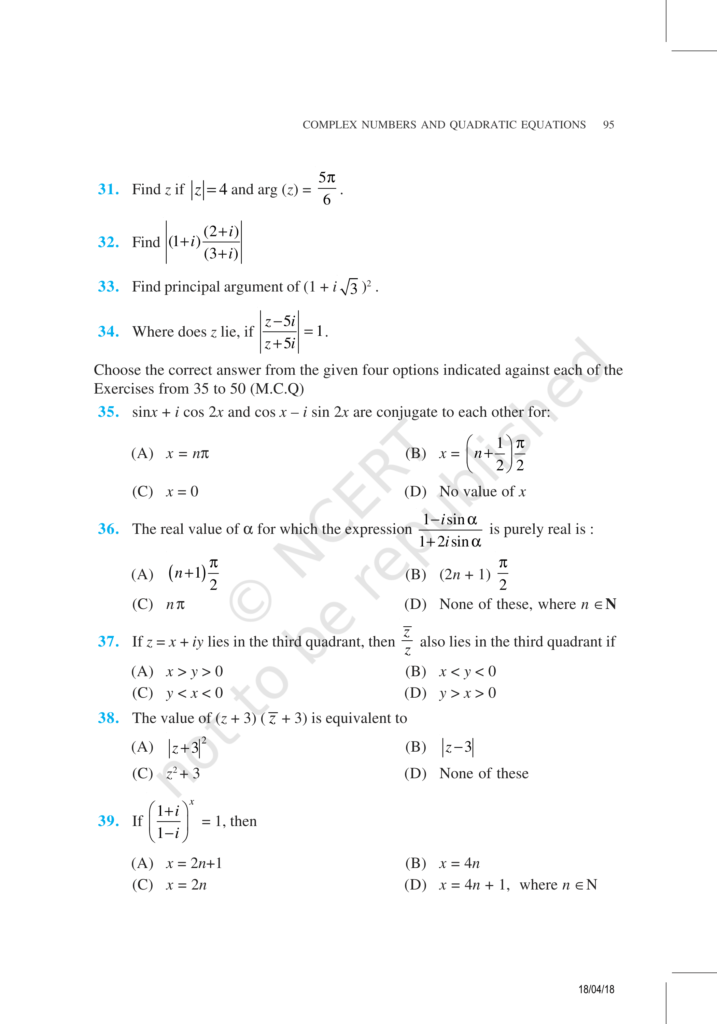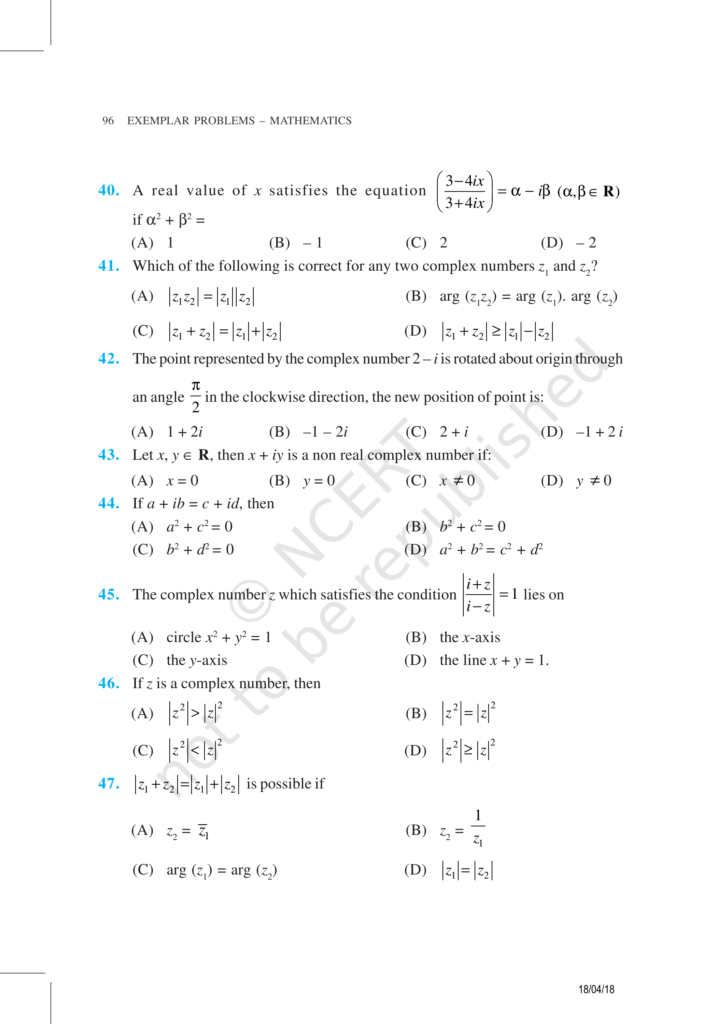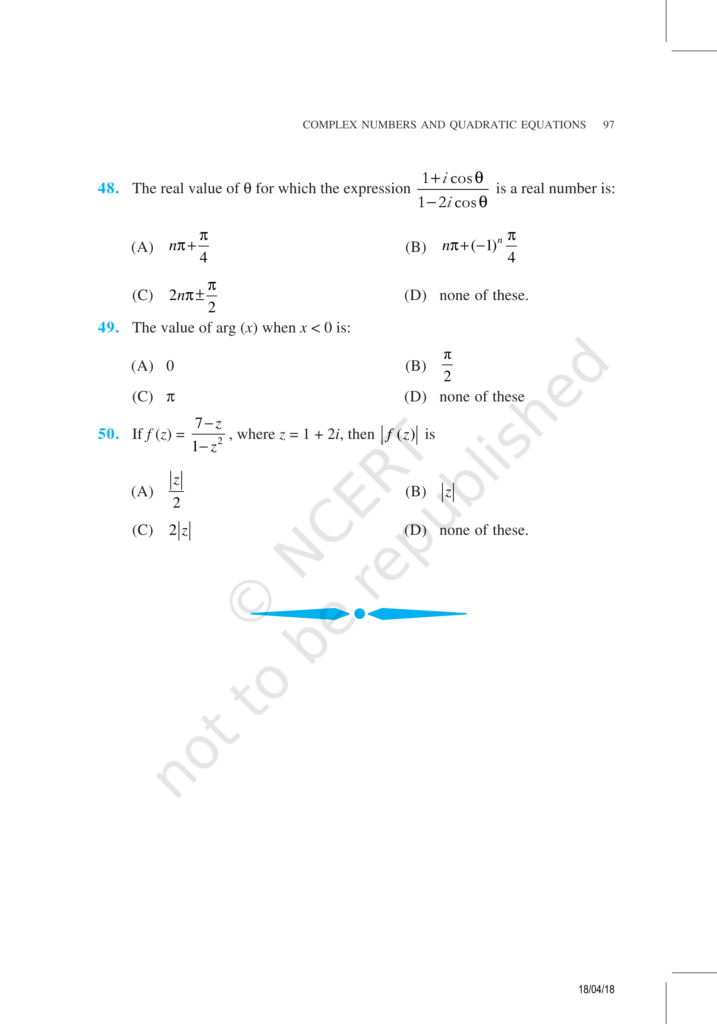Download the eSaral app and start learning from Kota's top IITians and doctors.

# NCERT Exemplar Class 11 Maths Chapter 5 - Complex Numbers and Quadratic Equations - Free PDF Download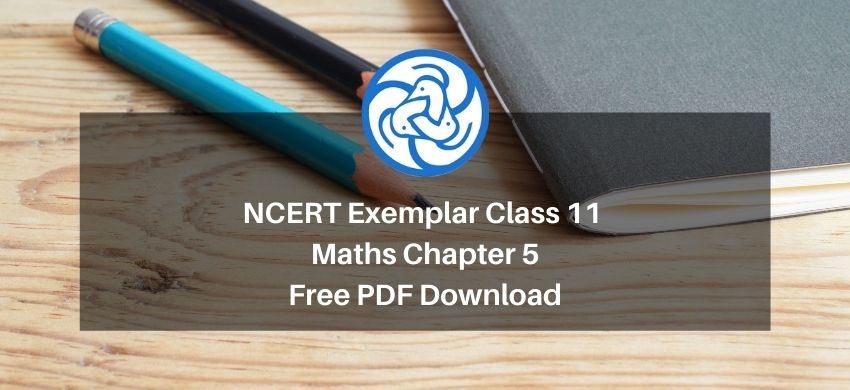Hey, are you a class 11 student? and looking for ways to download NCERT Exemplar Class 11 Maths Chapter 5 "Complex Numbers and Quadratic Equations"? If yes. Then read this post till the end.

If you want to score high in your Class 11 Maths exam then it is very important for you to understand and learn the topics of Class 11 Maths thoroughly.

So, without wasting more time let’s start.

### Download The PDF of NCERT Exemplars Class 11 Maths Chapter 5 "Complex Numbers and Quadratic Equations"